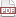### Tip 76: Bootstrap!

29 February 12. [link]PDF version

level: Reporting variances
purpose: Get a variance out of anything

Part of a series of tips on POSIX and C. Start from the tip intro page, or get 21st Century C, the book based on this series.

I haven't addressed the statisticians in a long time.

The bootstrap is a means of getting a variance out of data that has no right giving you a variance. For example, if you have only one data set, and you can calculate a single statistic from that data, how would you get the variance of that statistic?

The basic concept is the bootstrap principle, that if we sample from the data, then the resulting statistics behave as if we'd sampled from the population. So the bootstrap function will generate 1,000 samples from the data, measure the corresponding 1,000 statistics, and then report the covariance of those statistics. Good ol' Wikipedia has much more.

In terms of writing code, bootstrapping is the perfect application of a function that takes a function as input. Here's a worked example using Apophenia.

First, let's generate some data using a lopsided distribution:

apop_data *generate_a_sample(gsl_rng *r, int qty){
apop_model *lopsided =apop_model_set_parameters(apop_beta, 1, 3);

apop_data *full_sample= apop_data_alloc(qty);
for (int i=0; i<qty; i++)
apop_draw(&full_sample->vector->data[i], r, lopsided);
return full_sample;
}


Since I'm generating data myself, there's really no point in bootstrapping--I can just generate a thousand samples by calling this function a thousand times, and observe the variance. Bootstrapping really makes sense when you have exactly one data set on hand and need to make the most of it. So for the rest of this, just play along with the fiction that we don't know from where the data came.

Now we write a function to estimate some parameter. In this case, let's use the point that minimizes absolute cubed error. I really have little intuition about this (it weights extreme values heavily and seems like it'd be volatile, I suppose, but this turned out to not be all that true).

In fact, even solving for it would be annoying, so let's just use the optimizer. We write a model that has a log likelihood function that isn't really a log likelihood, but provides something for the optimizer to maximize:

double mqe_value(apop_data *d, apop_model *m){
double stat = m->parameters->vector->data; //alias this mess
double out=0;
for (int i=0; i< d->vector->size; i++)
out += fabs(gsl_pow_3(d->vector->data[i] - stat));
return -out; //Apophenia maximizes, but we want a minimum.
}

apop_model mqe = {.name="Minimize mean absolute cubed error",
.vbase=1, .log_likelihood=.mqe_value};


OK, now we have a data set and a model. Let's get ourselves some variances.

The bootstrap function will generate a bootstrap sample from the input sample, search for the optimum, and write that down. You can add the .keep_boots='y' option to the bootstrap call below to see what gets drawn. Then, the function reports the variance of all of the estimates it just did.

Here's all the code, including the above generation of toy data, the model to find the minimum mean cubed error, and the main that actually calls the bootstrap function. You've already met the first two parts (which I reprint for your cutting and pasting ease), and the main function should be easily readable, setting up the data and calling the one-liner that is the bootstrap function.

#include <apop.h>

apop_data *generate_a_sample(gsl_rng *r, int qty){
apop_model *lopsided =apop_model_set_parameters(apop_beta, 1, 3);

apop_data *full_sample= apop_data_alloc(qty);
for (int i=0; i<qty; i++)
apop_draw(&full_sample->vector->data[i], r, lopsided);
return full_sample;
}

double mqe_value(apop_data *d, apop_model *m){
double stat = m->parameters->vector->data; //alias this mess
double out=0;
for (int i=0; i< d->vector->size; i++)
out += fabs(gsl_pow_3(d->vector->data[i] - stat));
return -out; //Apophenia maximizes, but we want a minimum.
}

apop_model mqe = {.name="Minimize mean absolute cubed error",
.vbase=1, .log_likelihood=mqe_value};

int main(){
int qty=1e4;
gsl_rng *r = apop_rng_alloc(234);
apop_data *data = generate_a_sample(r, qty);
apop_data *boot_var = apop_bootstrap_cov(data, mqe);
printf("std error = %g\n", sqrt(boot_var->matrix->data));

//by the way, the actual best estimate:
apop_model *estimated = apop_estimate(data, mqe);
printf("estimate = %g\n", estimated->parameters->vector->data);
}